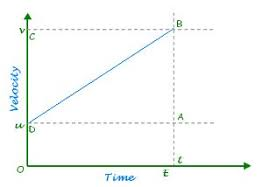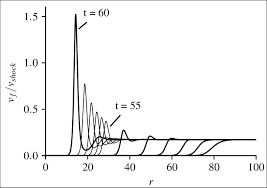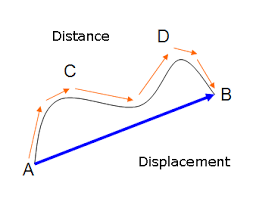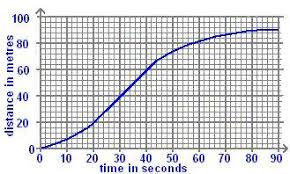## How to Calculate and Solve for Distance Covered | MotionThe image above represents distance covered.

To compute for distance covered, three essential parameters are needed and these parameters are initial velocity (u), acceleration (a) and time (t).

The formula for calculating distance covered:

S = ut + 0.5at²

Where;

S = Distance Covered
u = Initial Velocity
t = Time
a = Acceleration

Let’s solve an example;
Find the distance covered when the initial velocity is 21, acceleration is 10 and the time is 15.

This implies that;

u = Initial Velocity = 21
t = Time = 15
a = Acceleration = 10

S = ut + 0.5at²
S = (21 x 15) + (0.5 x 10 x 15²)
S = 315 + (0.5 x 10 x 225)
S = 315 + (1125)
S = 1440

Therefore, the distance covered is 1440 m .

Calculating the Initial Velocity when the Distance Covered, the Acceleration and the Time is Given.

u = S – 0.5at2 / t

Where;

u = Initial Velocity
S = Distance Covered
t = Time
a = Acceleration

Let’s solve an example;
Find the initial velocity when the distance covered is 48 with a time of 8 and an acceleration of 9.

This implies that;

S = Distance Covered = 48
t = Time = 8
a = Acceleration = 9

u = S – 0.5at2 / t
u = 48 – 0.5(9)(82) / 8
u = 48 – 0.5(9)(64) / 8
u = 48 – 288 / 8
u = – 240 / 8
u = – 30

Therefore, the initial velocity is – 30 m/s.

## How to Calculate and Solve for Final Velocity | MotionThe image above represents final velocity.

To compute for final velocity, three essential parameters are needed and these parameters are initial velocity (u), acceleration (a) and distance covered (S).

The formula for calculating final velocity:

v = √(u² + 2as)

Where;

v = Final Velocity
u = Initial Velocity
a = Acceleration
s = Distance Covered

Let’s solve an example;
Find the final velocity when the initial velocity is 13, acceleration is 9.8 and the distance covered is 32.

This implies that;

u = Initial Velocity = 13
a = Acceleration = 9.8
s = Distance Covered = 32

v = √(u² + 2as)
v = √(13² + (2 x 9.8 x 32))
v = √(169 + (627.2))
v = √(796.2)
v = 28.21

Therefore, the final velocity is 28.21 m/s.

Calculating the Initial Velocity when the Final Velocity, the Acceleration and the Distance Covered is Given.

u = √v² – 2as

Where;

u = Initial Velocity
v = Final Velocity
a = Acceleration
s = Distance Covered

Let’s solve an example;
Find the initial velocity when the final velocity is 30, the acceleration is 8 and the distance covered is 11.

This implies that;

v = Final Velocity = 30
a = Acceleration = 8
s = Distance Covered = 11

u = √v² – 2as
u = √30² – 2(8 x 11)
u = √900 – 2(88)
u = √900 – 176
u = √724
u = 26.90

Therefore, the initial velocity is 26.90 m/s.

## How to Calculate and Solve for Distance Covered | MotionThe image above represents distance covered.

To compute for distance covered, two essential parameters are needed and these parameters are angular displacement (θ) and radius (r).

The formula for calculating distance covered:

S = rθ

Where;

S = Distance Covered
θ = Angular Displacement

Lets’s solve an example;
Find the distance covered when the angular displacement is 22 and the radius is 14.

This implies that;

θ = Angular Displacement = 22

S = rθ
S = 6 x 16
S = 96

Therefore, the distance covered is 96 m.

Calculating the Radius when the Distance Covered and the Angular Displacement is Given.

r = S / θ

Where;

S = Distance Covered
θ = Angular Displacement

Lets’s solve an example;
Find the radius when the distance covered is 70 and the angular displacement is 30.

This implies that;

S = Distance Covered = 70
θ = Angular Displacement = 30

r = S / θ
r = 70 / 30
r = 2.3

## How to Calculate and Solve for Distance Covered | MotionThe image above represents distance covered.

To compute for distance covered, three essential parameters are needed and these parameters are final velocity (v), initial velocity (u) and time (t).

The formula for calculating distance covered:

S = ((u + v) / 2) t

Where;

S = Distance Covered
u = Initial Velocity
v = Final Velocity
t = Time

Let’s solve an example;
Find the distance covered when the initial velocity is 6, final velocity is 11 and time is 14.

This implies that;

u = Initial Velocity = 6
v = Final Velocity = 11
t = Time = 14

S = ((u + v) / 2) t
S = ((6 + 11) / 2) 14
S = ((17) / 2) 14
S = (8.5) 14
S = 119

Therefore, the distance covered is 119 m.

Calculating the Initial Velocity when the Distance Covered, the Final Velocity and the Time is Given.

u = (2S / t) – v

Where;

u = Initial Velocity
S = Distance Covered
v = Final Velocity
t = Time

Let’s solve an example;
Find the initial velocity when the distance covered is 48, the final velocity is 20 and the time is 4.

This implies that;

S = Distance Covered = 48
v = Final Velocity = 20
t = Time = 4

u = (2S / t) – v
u = (2(48) / 4) – 20
u = (96 / 4) – 20
u = 24 – 20
u = 4

Therefore, the initial velocity is 4 m/s.